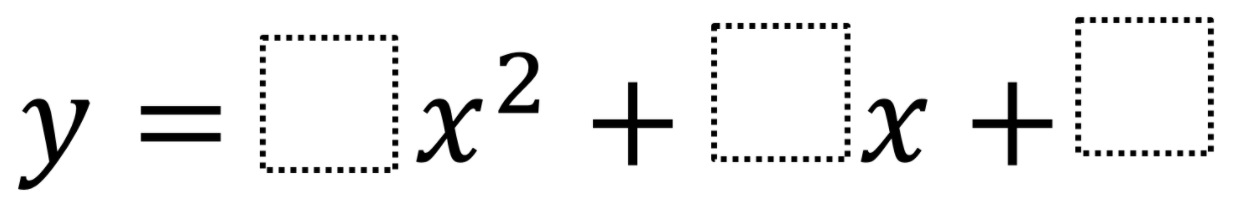# Parabola’s Vertex

Directions: Using the digits 1 to 9 at most one time each, place a digit in each box to create a correct sentence: The vertex of the parabola, y = ▢ x² + ▢ x + ▢, lies on the horizontal axis### Hint

The vertex of the parabola y = ax² + bx + c lies on the horizontal axis if and only if the equation ax² + bx + c =0 has an unique solution (b² = 4ac)